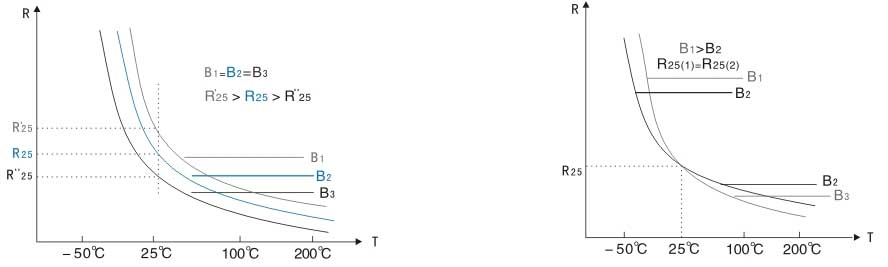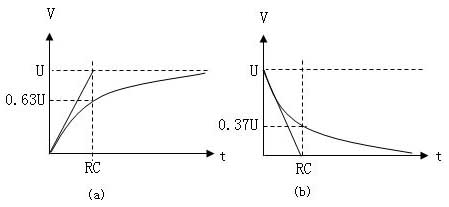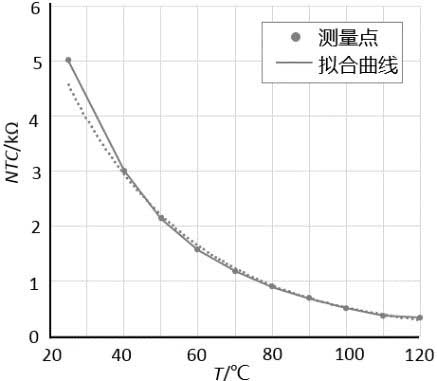﻿ Main Technical Parameters of NTC Thermistor_

China sensor manufacturers

# Main Technical Parameters of NTC Thermistor

Key words: thermistor, NTC thermistor, zero power resistance value RT, zero power resistance R25, B value, dissipation coefficient δ, thermal time constant τ, rated power Pr, resistance-temperature characteristics

NTC thermistor is a kind of semiconductor ceramic module which is sintered at high temperature with transition metal oxide as the main raw material. It has a very large negative temperature coefficient, and the resistance value changes with the ambient temperature or self-heating due to the passing current. That is, at a certain measured power, the resistance value rapidly decreases as the temperature rises. Using this characteristic, the temperature of NTC thermistor can be determined by measuring its resistance value, so as to achieve the purpose of temperature detection and control.

1. Zero power resistance value RT
At the specified temperature, the resistance value measured by the measured power that causes the resistance change to be negligible with respect to the total measurement error is used.
2, zero power resistor R25
Refers to the zero power resistance measured at 25 °C. Unless otherwise specified, it is the design resistance of the thermistor and the nominal resistance.
3, B value
The B value is the thermal index of the negative temperature coefficient thermistor. It is defined as the ratio of the difference between the natural logarithm of zero power resistance at two temperatures and the difference between the reciprocal of two temperatures.RT1--Zero power resistance at temperature T1
RT2--Zero power resistance at temperature T2
T1=2731.5k +( T1℃), T2=2731.5k+ (T2℃)

Unless otherwise stated, the B value is calculated from the zero power resistance values of 25 ° C (298.15 K) and 50 ° C (323.15 K), and the B value is not a strict constant over the operating temperature range.

4, dissipation coefficient δ
δ=ΔP/ΔT (mW/°C)
That is: the ratio of the rate of change of the thermistor's dissipated power to its corresponding temperature change at a specified ambient temperature. It represents the power required to raise the thermal resistor by 1 °C. In the operating temperature range, δ varies with ambient temperature.5, thermal time constant τ
Under zero power condition, when the temperature changes abruptly, the temperature of thermistor body changes 63.2% of the time required for the difference between initial and final temperature. τ is proportional to the thermal capacity C of the thermistor and inversely proportional to its dissipation coefficient δ, namely:
τ=C/δ

6, rated power Pr
Rated power = dissipation factor δ × (maximum operating temperature Tmax - 25 ° C)

7, resistance-temperature characteristics
The law of the resistance of the thermistor varies with temperature, which is roughly as follows:R1: resistance at absolute temperature T1 (K) of
B : B constant T(K)=T(°C)+273.15

﻿
﻿